﻿ 动态规划系列之六01背包问题_其他_开心洋葱网
• 欢迎访问开心洋葱网站，在线教程，推荐使用最新版火狐浏览器和Chrome浏览器访问本网站，欢迎加入开心洋葱` QQ群`
• 为方便开心洋葱网用户，开心洋葱官网已经开启复制功能！
• 欢迎访问开心洋葱网站，手机也能访问哦~欢迎加入开心洋葱多维思维学习平台` QQ群`
• 如果您觉得本站非常有看点，那么赶紧使用Ctrl+D 收藏开心洋葱吧~~~~~~~~~~~~~！
• 由于近期流量激增，小站的ECS没能经的起亲们的访问，本站依然没有盈利，如果各位看如果觉着文字不错，还请看官给小站打个赏~~~~~~~~~~~~~！

# 动态规划系列之六01背包问题

1562次浏览# 问题

A `重 2 价值为 2`
B `重 3 价值为 6`
C `重 4 价值为 4`
D `重 4 价值为 5`
E `重 1 价值为 3`

# 原理

``````0 0 0 0 0 0 0 0 0 0 0 0
``````

• 假设变量 `j` 为背包的容量，`i`表示第i个物品，weight[i]表示`i`物品的重量， value[i]表示`i`物品的价值,bag[i][j]表示在放入第i个物品时，背包容量为j时的背包价值。那么会有一个表达式用于选择：

• 如果背包容量`j`小于物品i的重量，放不进物品，背包的价值等于之前物品放入的价值。所以：`bag[i][j] = bag[i-1][j]`
如果背包的容量`j`大于物品i的重量，能够放入物品，那么要不要放则取决于背包放入物品时价值大还是不放入价值大所以： `bag[i][j] = max(bag[i-1][j-weight[i]] + value[i] , bag[i-1][j])`

`为什么是前一行?`

## 不放入任何物品时，背包承重从0到10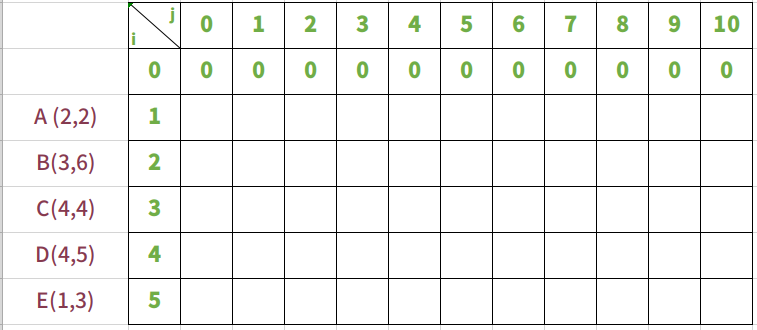## 放入物品A，背包承重从0到10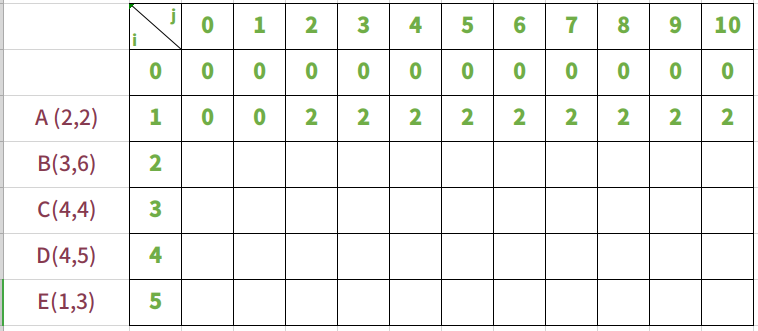## 放入物品B，背包承重从0到10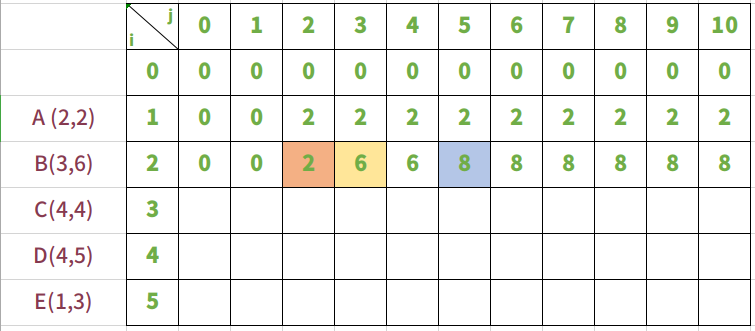`i=2,j=2`, j还不能放入物品B，所以`bag=bag[i-1][j]``bag=2`
`i=2,j=3`, j刚好能放入物品B，所以`bag= max(bag[i-1][j-weight[i]] + value[i] , bag[i-1][j])`,即`bag = max(bag+6,bag)`==>`bag = max(6,2)`。所以`bag = 6` 。放入物品B价值大
`i=2,j=4`, j能放入物品B，所以同上`bag = max(bag+6,bag)` ==> `bag = max(6,2)`。所以`bag = 6`。放入物品B价值大
`i=2,j=5`, j能放入物品B，所以`bag = max(bag+6,bag)` ==> `bag = max(8,2)`。所以`bag = 8`。放入物品B价值大。在背包重量j=5时，能够先放入B，然后再放入A。
`i=2,j=6`, 同上
`i=2,j=7`, 同上
`i=2,j=8`, 同上
`i=2,j=9`, 同上
`i=2,j=10`, 同上

## 放入物品C，背包承重从0到10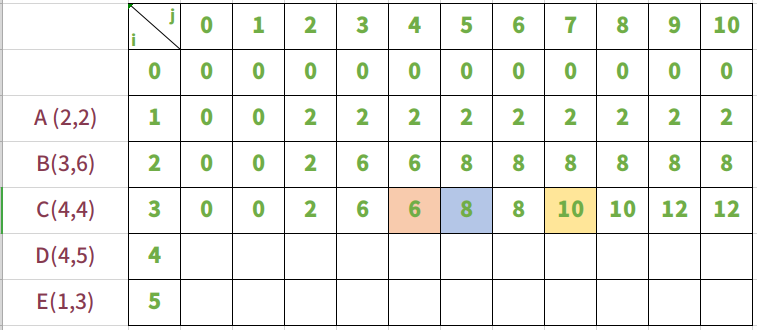`i=4,j=4` ,背包能够放入物品C。`bag = max(bag[4-1][4-4]+4,bag[4-1])` ==> `bag = max(4,6)`。所以`bag = 6`。这里不再是放入物品C背包价值大，而是不放入时价值更大。
`i=4,j=5` ,背包能够放入物品C。`bag = max(bag[4-1][5-4]+4,bag[4-1])` ==> `bag = max(4,6)`。所以`bag = 6`。同样，这里不放入物品C背包价值更大。
`i=4,j=7` , 背包能够放入物品C。`bag = max(bag[4-1][7-4]+4,bag[4-1])` ==> `bag = max(6+4,8)`。所以`bag = 10`。这里放入物品C背包价值更大。

## 放入物品D，背包承重从0到10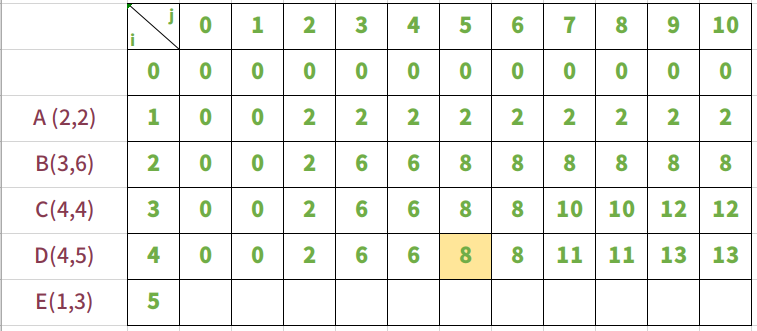i=4,j=5,背包能够放入物品D，`bag = max(bag[4-1][5-5]+4,bag[4-1])` ==> `bag = max(4,8)`。所以`bag = 8`。同样，这里不放入物品D背包价值大。

## 放入物品E，背包承重从0到10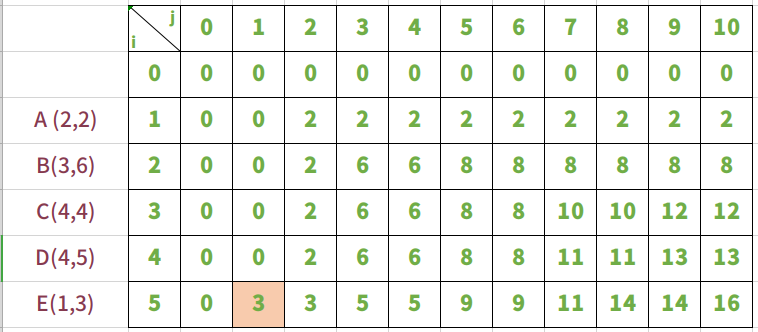i=5,j=1,背包刚好能够放入物品E。这是`bag = max(bag[5-1][1-1]+3,bag[5-1])` ==> `bag = max(3,0)`。所以`bag = 3`。这是放入物品E背包价值大

## 结果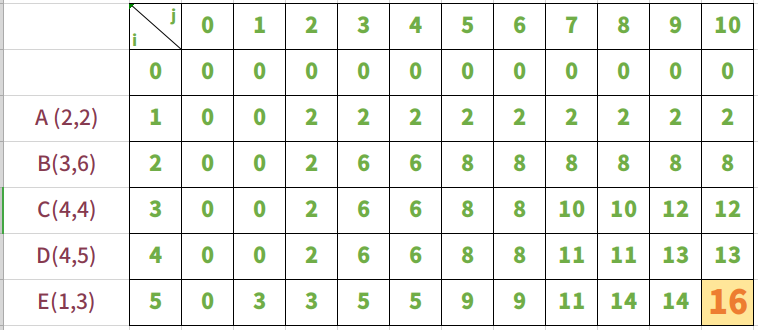# 代码实现

## 背包最大价值

``````
weight = [0,2,3,4,4,1]
value = [0,2,6,4,5,3]
weight_most=10

bag = [[0 for i in range(weight_most+1)] for j in range(len(weight))]

for i in range(1,len(weight)):
for j in range(1,weight_most+1):
if j >= weight[i]:
bag[i][j] = max(bag[i-1][j-weight[i]]+value[i],bag[i-1][j])
else:
bag[i][j] = bag[i-1][j]

print(bag[-1][-1])
``````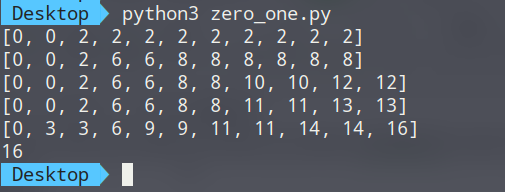## 找到背包里的物品

``````weight = [2,3,4,4,1]
value = [2,6,4,5,3]
weight_most=10

dp =  * (weight_most+1)

for i in range(len(weight)):
for j in range(weight_most,weight[i]-1,-1):
dp[j] = max(dp[j-weight[i]]+value[i], dp[j])

print(dp)
``````

## 01背包问题优化

01背包的时间复杂度已经无法优化，但是在空间复杂度上还存在优化的可能。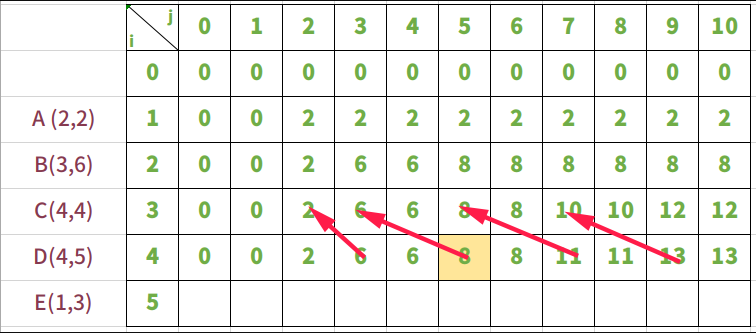``````weight = [0,2,3,4,4,1]
value = [0,2,6,4,5,3]

weight_most=10

# 使用一维数组完成记录
bag = [0 for i in range(weight_most+1)]
for i in range(1,len(weight)):
# 从后向前遍历，因为后面的值依赖前面的值，从后向前不会破坏前面的值，就不会破坏后面的依赖
for j in range(weight_most,0,-1):
if j >= weight[i]:
bag[j] = max(bag[j-weight[i]]+value[i],bag[j])
print(bag[-1])
``````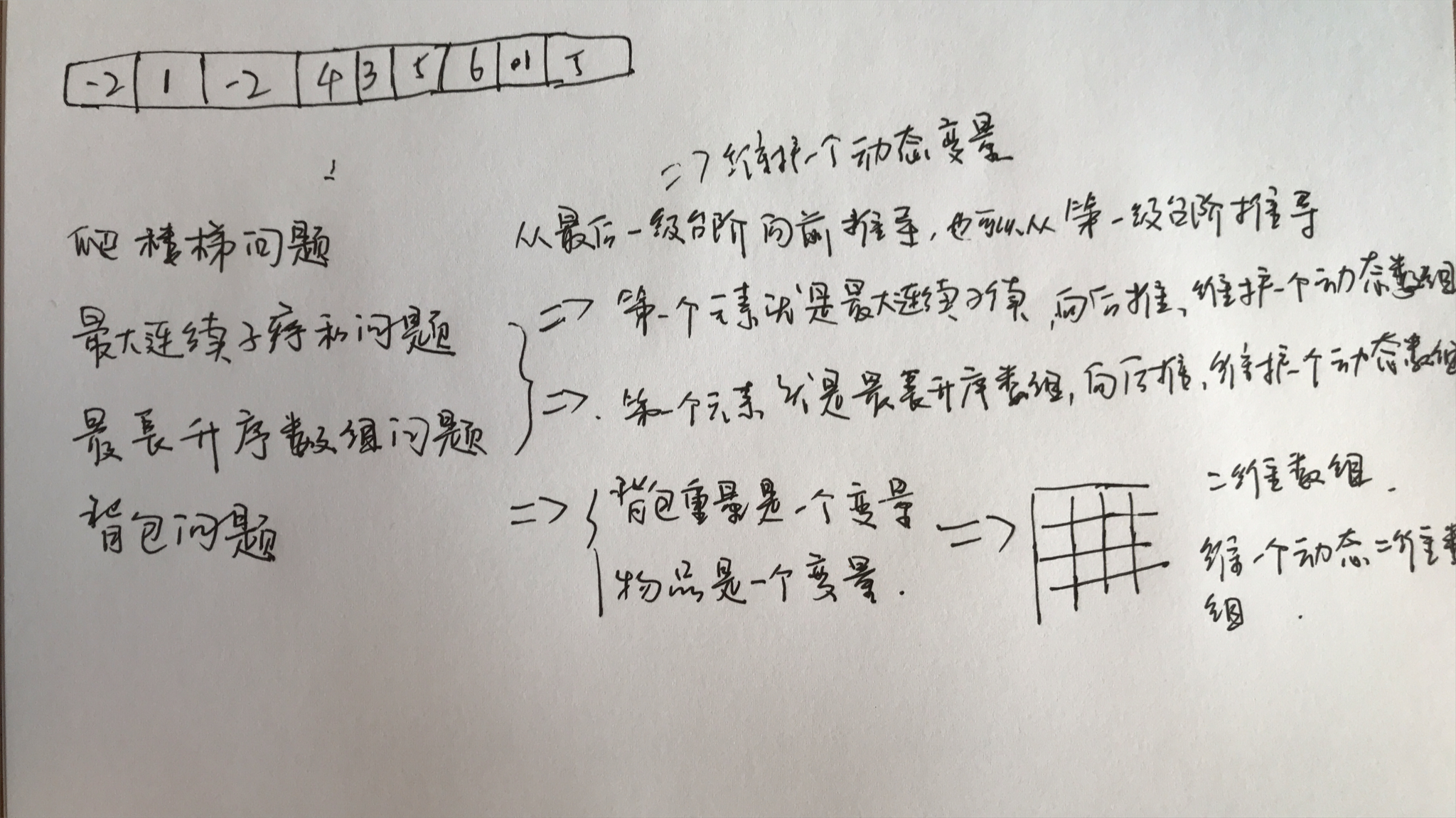# 贪心算法

`过程`

`特征`

## DP思想：

1、把一个大的问题分解成一个一个的子问题。
2、如果得到了这些子问题的解，然后经过一定的处理，就可以得到原问题的解。
3、如果这些子问题与原问题有着结构相同，即小问题还可以继续的分解。
4、这样一直把大的问题一直分下去，问题的规模不断地减小，直到子问题小到不能再小，最终会得到最小子问题。
5、最小子问题的解显而易见，这样递推回去，就可以得到原问题的解。

## 贪心算法：

• 对其作出一个选择后，只剩下一个子问题需要求解。
• 证明做出贪心选择后，原问题总是存在最优解，即贪心选择总是安全的。
• 剩余子问题的最优解与贪心选择组合即可得到原问题的最优解。

## 能使用动态规划算法的条件：

• 版权声明

本站的文章和资源来自互联网或者站长
的原创，按照 CC BY -NC -SA 3.0 CN
协议发布和共享，转载或引用本站文章
应遵循相同协议。如果有侵犯版权的资
源请尽快联系站长，我们会在24h内删
除有争议的资源。
• 合作网站

• 友情链接

• 关于我们

一群热爱思考，热爱生活，有理想的新社会主义接班人的多维思维学习平台，天行健，君子以自强不息。地势坤，君子以厚德载物。